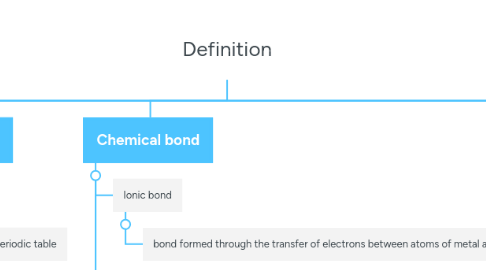# DefinitionSabrina Marwan
Get Started. It's FreeDefinition## 1. The structure of atom

### 1.2. Atom

1.2.1. The smallest particles of an element that can participate in a chemical reaction

1.3.1. New node

### 1.7. Nucleon number

1.7.1. The nucleon number of an element is the total number of protons and neutrons in its atom

## 2. Periodic Table of Elements

### 2.1. Group

2.1.1. The vertical column in the periodic table

## 3. Chemical Formulae and equation

### 3.1. Relative atomic mass

3.1.1. The average mass of one atom of an element when compared with 1/12 of the mass of an atom carbon-12

### 3.2. Relative molecular mass

3.2.1. The average mass of one molecule when compared with 1/12 of the mass of an atom carbon-12

### 3.3. Relative formula mass

3.3.1. The average mass of one formula unit of the ionic substance when compared with 1/12 of the mass of atom carbon-12

### 3.4. Molar mass

3.4.1. The mass of 1 mol of the substance with the unit grams per mol or g mol -3

### 3.7. Chemical formula

3.7.1. A representation of a chemical substance using letters for atoms and subscript numbers to show the numbers of each type of atoms that are present in substance.

### 3.8. Empirical formula

3.8.1. the simplest whole number ratio of atom of each element in a compound

### 3.10. Chemical equationj

3.10.1. Representation of a chemical reaction in words or using chemicals formulas

## 4. Chemical bond

### 4.1. Ionic bond

4.1.1. bond formed through the transfer of electrons between atoms of metal and non-metal to achieve the stable octet electron arrangement.

### 4.2. Ionic compound

4.2.1. consist of positive ions and negative ions which are held by strong electrostatic forces of attraction.

### 4.3. Covalent compound

4.3.1. neutral molecules which are held by weak intermolecular forces (Van der Waals).

## 5. Electrochemistry

### 5.1. Electrolytes

5.1.1. Substance in molten state or aqueous solution that can conduct electricity due to the presence of free moving ions

### 5.2. Non-electrolytes

5.2.1. Substance that cannot conduct electricity in molten state or aqueous solution

### 5.3. Electrolysis

5.3.1. A reaction that takes place when electricity passes through an electrolyte

### 5.5. Purification of metals

5.5.1. The process of obtaining a pure metal from an impure metal through electrolysis.

### 5.6. Electroplating of metals

5.6.1. The process of coating a layer of metal onto another metal using electrolysis.

### 5.7. Simple voltaic cell

5.7.1. The process of coating a layer of metal onto another metal using electrolysis.

### 5.8. Displacement reaction

5.8.1. A reaction where a more electropositive metal displace another metal from its salt solution.

## 6. Acids and basess

### 6.2. Acid

6.2.1. chemical substance which ionizes in water to produce hydrogen ions, H+

### 6.4. pH value

6.4.1. measure of the concentration of hydrogen ions, H+.

### 6.5. Strong alkali

6.5.1. ionises (dissociates) completely in water to form hydroxide ions, OH- of high concentration.

### 6.6. Weak alkali

6.6.1. ionises (dissociates) partially in water to form hydroxide ions, OH- of low concentration.

### 6.7. Neutralization

6.7.1. A reaction between an acid and an alkali or a base to produce salt